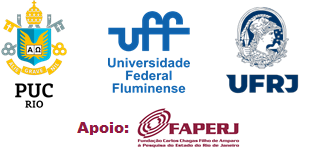Regularity of Lyapunov exponents
Carlos H. Vasquez

Let us consider a C ∞ – one parameter family of C ∞ diffeomorphisms f t , t ∈ I , defined on a compact orientable Riemannian manifold M . If the family admits a Df t –invariant subbundle E ( t ) and an invariant probability measure μ for every t ∈ I , then the integrated Lyapunov exponent λ ( t ) of f t over E ( t ) is well defined. In this talk we discuss about the regularity of the function λ ( t ) . The explicit expansion of λ ( t ) , involves the regularity of the family, regularity of the splitting and the smooth vector field X on M , which is tangent to the family f − 1 0 ◦ f t in t = 0 . Within the context of dominated splittings. We conjecture that if the 2 bundles of the splitting are of class C α and respectively C β for f 0 , then the integrated Lyapunov exponent λ ( t ) of each bundle has expansion of order ( α + β ) in the parameter t at t = 0 . We prove the conjecture in some cases, using some tools that may have their own interest. Finally, if the time allows it, we discuss about some possible applications of the previous results including the case of variable measure, the abundance of non–uniform hyperbolicity, the abundance of non–absolute continuous central and intermediate foliations, regularity of metric entropy and rigidity of Anosov maps on surfaces. Work in progress joint with Radu Saghin and Pancho Valenzuela-Henríquez.